## Exercises

1. Suppose that a very fast path planning algorithm runs on board of a mobile robot (for example, it may find an answer in a few milliseconds, which is reasonable using trapezoidal decomposition in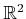). Explain how this method can be used to simulate having a feedback plan on the robot. Explain the issues and trade-offs between having a fast on-line algorithm that computes open-loop plans vs. a better off-line algorithm that computes a feedback plan.
2. Use Dijkstra's algorithm to construct navigation functions on a 2D grid with obstacles. Experiment with adding a penalty to the cost functional for getting too close to obstacles.
3. If there are alternative routes, the NF2 algorithm does not necessarily send the state along the route that has the largest maximum clearance. Fix the NF2 algorithm so that it addresses this problem.
4. Tangent space problems:
1. For the manifold of unit quaternions, find basis vectors for the tangent space in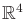at any point.
2. Find basis vectors for the tangent space in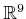, assuming that matrices in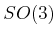are parameterized with quaternions, as shown in (4.20).
5. Extend the algorithm described in Section 8.4.3 to make it work for polygons that have holes. See Example 8.16 for a similar problem.
6. Give a complete algorithm that uses the vertical cell decomposition for a polygonal obstacle region into construct a vector field that serves as a feedback plan. The vector field may be discontinuous.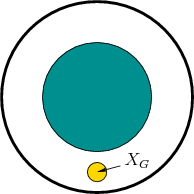7. Figure 8.23 depicts a 2D example for which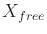is an open annulus. Consider designing a vector field for which all integral curves flow into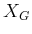and the vector field is continuous outside of. Either give a vector field that achieves this or explain why it is not possible.
8. Use the maximum-clearance roadmap idea from Section 6.2.3 to define a cell decomposition and feedback motion plan (vector field) that maximizes clearance. The vector field may be discontinuous.
9. Develop an algorithm that computes an exact cover for a polygonal configuration space and ensures that if two neighborhoods intersect, then their intersection always contains an open set (i.e., the overlap region is two-dimensional). The neighborhoods in the cover should be polygonal.
10. Using a distance measurement and Euler angles, determine the expression for a collision-free ball that can be inferred (make the ball as large as possible). This should generalize (8.54).
11. Using a distance measurement and quaternions, determine the expression for a collision-free ball (once again, make it as large as possible).
12. Generalize the multi-linear interpolation scheme in (8.59) from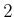todimensions.
13. Explain the convergence problems for value iteration that can result if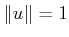is used to constraint the set of allowable actions, instead of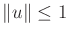.

Implementations

14. Experiment with numerical methods for solving the function (8.49) in two dimensions under various boundary conditions. Report on the efficiency and accuracy of the methods. How well can they be applied in higher dimensions?
15. Implement value iteration with interpolation (it is not necessary to use the method in Figure 8.20) for a polygonal robot that translates and rotates among polygonal obstacles in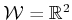. Define the cost functional so that the distance traveled is obtained with respect to a weighted Euclidean metric (the weights that compare rotation to translation can be set arbitrarily).
16. Evaluate the efficiency of the interpolation method shown in Figure 8.20 applied to multi-linear interpolation given by generalizing (8.59) as in Exercise 12. You do not need to implement the full value iteration approach (alternatively, this could be done, which provides a better comparison of the overall performance).
17. Implement the method of Section 8.4.2 of computing vector fields on a triangulation. For given input polygons, have your program draw a needle diagram of the computed vector field. Determine how fast the vector field can be recomputed as the goal changes.
2. Extend the algorithm and implementation to the case in which there are polygonal holes in.
20. Try to experimentally construct navigation functions by adding potential functions that repel the state away from obstacles and attract the state toward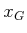. For simplicity, you may assume that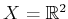and the obstacles are discs. Start with a single disc and then gradually construct more complicated obstacle regions. How difficult is it to ensure that the resulting potential function has no local minima outside of?